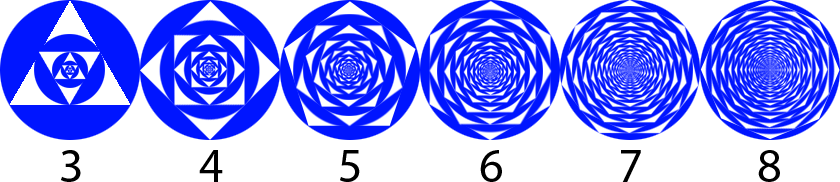# Cyclic Regular Polygon Fractals

Calculus Level 3The $n^{\text{th}}$ figure in the above sequence is constructed by the following procedure:

1. Draw a blue disc of radius $\displaystyle\sqrt{\frac{2016}{\pi}}$
2. Remove a regular $n$-gon area from the (smallest) disc
3. Inscribe a blue disc inside the empty $n$-gon space
4. Repeat steps 2-4

Let $A_n$ be the total blue area of the $n^{\text{th}}$ figure in the sequence.

Compute $\displaystyle\lim_{n\to\infty}A_n$.

×